TLDR; When used inside of a filter context, CALCULATE performs context transition, transforming the current row context into a filter context. We explore how context transition conditions the output of calculated columns in tables, and how measures and relationships interact with it.

So far in our Evaluation Contexts in DAX series we have learned about the row and filter contexts, how these affect the output of our DAX code, and how they interact with different types of relationships between tables in our data model. In this third and final part of this series, we learn about how `CALCULATE` performs Context Transition when used inside of a filter context.

## What happens when we don’t use `CALCULATE`

Let’s revisit once more our example with a data model containing data on the sales of certain products from a store. The two tables we will be using are the Product table, containing details on the products available in the store, and the Sales table, containing details on the sales made.

Let’s see what happens when Context Transition doesn’t happen.

The following DAX formula will display the sum of all the unit prices in the Product table visible in the current filter context.

`Total Unit Price = SUM ( 'Product'[Unit Price] )`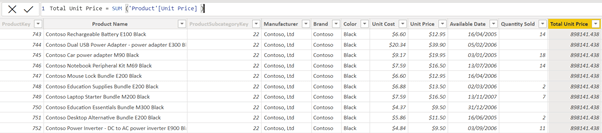One easy mistake to make is to expect the Total Unit Price column to be equal to the Unit Price column. It is true that when we create a calculated column in a table, there is an automatic row context for the product table. However, what `SUM` does is aggregate all the values in the current filter context and display their sum. Because the filter context is empty, no filters are being applied to the table The Total Unit Price calculated column returns the sum of all cells in the Unit Price column, showing the same value in all cells of the column.

## How CALCULATE introduces Context Transition

`CALCULATE` performs context transition, meaning it transforms the row context into a filter context.

When `CALCULATE` is used inside of a row context, like in a calculated column, it turns the current row context into a filter context, deleting the current row context. Anything inside of `CALCULATE` will be computed in a filter context containing only one row. Let’s see how this works.

Let’s create a new calculated column like before, except this time we will wrap our `SUM` inside of `CALCULATE`.

`Total Unit Price CALCULATE = CALCULATE ( SUM ( 'Product'[Unit Price] ) )`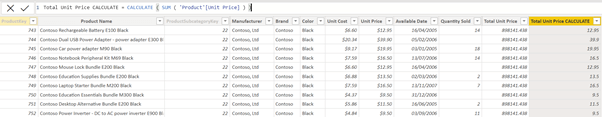Note that the output of our new calculated column using `CALCULATE` is identical to the Unit Price column. This is because as the row context iterates through the rows of the table, that row context becomes a filter context. As we have seen before, `SUM` aggregates all the values in the current filter context and sums them. This time, the filter context contains only one row at a time, so the sum will be the value itself.

## CALCULATE and Measures

One important thing to understand about measures is that they are automatically surrounded by `CALCULATE`. In our previous example this would mean that if we created a calculated column where Total Unit Price is a measure, this column would yield the same result as our Total Unit Price `CALCULATE` column.

In order to see this in action, let’s first create a measure that calculates the sum of the Unit Price column in the Product table.

`Total_Unit_Price = SUM ('Product'[Unit Price] )`

This is the same formula as in the Total Unit Price calculated column we had before, only this is a measure now.

Now, we create a calculated column from this measure.

`Total Unit Price Measure = 'Product'[Total_Unit_Price]`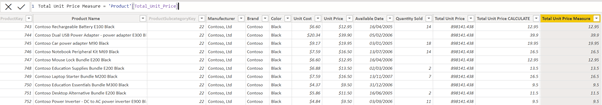We see how the new calculated column is identical to the one computed using `CALCULATE` (and to the original Unit Price column, for that matter), because `CALCULATE` is implied when a measure is used inside of a calculated column.

## Context Transition and Table Relationships

In our last post, we explained how different types of relationships between tables in the data model affect the output of our DAX code. So how does context transition interact with relationships? The answer is just as you would expect.

Here’s a little reminder of how evaluation contexts propagate through relationships:

• Row contexts never propagate through relationships.
• Filter contexts do propagate through relationships, but only from the one side to the many side of the relationship.

In our data model, the Product table is on the many side of a one-to-many relationship with the Sales table. This means the only the filter context is propagated from the Product to the Sales table.

Our aim is to investigate whether context transition happens between tables.

In the Product table, let’s add a column that computes the sum of the Sales Amount in the Sales table.

`Total Sales Amount = SUM ( Sales[Sales Amount] )`

This will give the sum of all rows in the Sales Amount column visible in the current filter context. We know also that the row context does not propagate through relationships. So, because the filter context is currently empty, all the cells in the new calculated column will display the same value – the sum of all the rows in the Sales Amount column.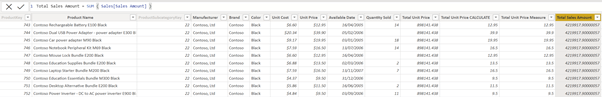Just like we did before, let’s surround our formula with `CALCULATE`. We now create a new calculated column with the following formula:

`Total Sales Amount CALCULATE = CALCULATE ( SUM ( Sales[Sales Amount] ) )`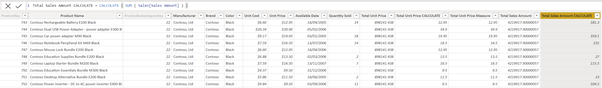And, yes, you guessed it. `CALCULATE` performs context transition between relationships as well. Why is that? Because filter contexts propagate through relationships, from the one side to the many side. Here, every time `CALCULATE` iterates through the row context in the Product table, this row context is transformed into a filter context, which is then propagated onto the Sales table. The Sales table is then only going to show the rows corresponding to the one row visible at that point in the Product table. All the cells visible in that filter context in the Sales Amount column are added and the result shows in the Product table.

## Conclusion

Context Transition is a powerful feature of `CALCULATE`, but it can create confusion sometimes. The two main things to remember are:

• When used inside of a row context, `CALCULATE` will transform that row context into a filter context.
• Measures are automatically surrounded by `CALCULATE`

After that, knowing the rules about how filter contexts work and propagate through relationships, it becomes easier to understand and predict the result of our DAX code.

### Elisenda Gascon

#### Apprentice Engineer IIElisenda was an Apprentice Engineer from 2021 to 2023 at endjin after graduating with a mathematics degree from UCL. Her passion for problem solving drove her to pursue a career in software engineering.

During her time at endjin, she helped clients by delivering data analytics solutions, using tools such as Azure Synapse, Databricks notebooks, and Power BI. Through this, she also gained experience as a consultant by delivering presentations, running customer workshops, and managing stakeholders.

Through her contributions to internal projects, she gaines experience in web development in ASP.NET, contributed to the documentation of the Z3.Linq library, and formed part of a team to develop a report to explore global trends in wealth & health.

During her training as a software engineer, Elisenda wrote a number of blog posts on a wide range of topics, such as DAX, debugging NuGet packages, and dependency injection. She has also become a Microsoft certified Power BI analyst and obtained the Green Software for Practitioners certification from the Linux Foundation.